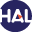Home page > 06. Volume 3 N°2 (2006) > Error estimate for Finite volume approximate solutions of oblique (...)

# Error estimate for Finite volume approximate solutions of oblique derivative boundary value problem

Sunday 6 December 2009,

Full Title: Error estimate for Finite volume approximate solutions of some oblique derivative boundary value problems

Abstract:

This paper is an improvment of [BG 05], concerning the Laplace equation with an oblique boundary condition. When the boundary condition involves a regular coefficient, we present a weak formulation of the problem and we prove some existence and uniqueness results of the weak solution. We develop a Finite volume scheme and we prove the convergence of the Finite volume solution to the weak solution, when the mesh size goes to zero. We also present some partial results for the interesting case of a discontinuous coefficient in the boundary condition. In particular, we give a Finite volume scheme, taking in consideration the discontinuities of this coefficient Finally, we obtain some error estimates (in a convenient norm) of order ph (where h is the mesh size), when the solution u is regular enough.

Paper presented by:

Prof. F. BenkhaldounVoir sur HAL : https://hal.archives-ouvertes.fr/hal-01114201